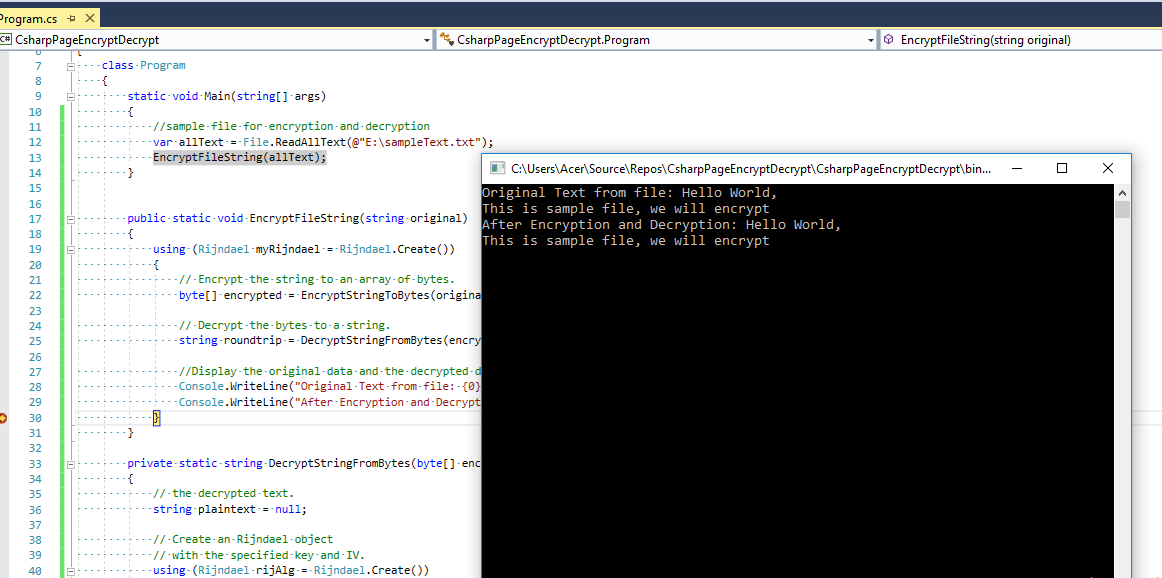In one of the previous article, I have mentioned how to Encrypt & decrypt password (C# Console application example), now in this article, I have mentioned how to encrypt and decrypt files in C#. We can have two easy method to encrypt/decrypt files, one using Cryptography and another is using FileInfo.Encrypt() method, let's take a look at both of the methods.

### Encrypt/Decrypt file using Cryptography Rijndael Class in C#

For this, we will create a new Console application in Visual Studio, so navigate to File-> New -> Project -> Select "Windows Desktop" from left pane and select "Console application" from right-pane, name your project and Click "Ok"

In the program.cs, you can use the below code, I have commented out most of the code, to understand the logic and execution of the code.

``````using System;
using System.IO;
using System.Security.Cryptography;

namespace CsharpPageEncryptDecrypt
{
class Program
{
static void Main(string[] args)
{
//sample file for encryption and decryption
EncryptFileString(allText);
}

public static void EncryptFileString(string original)
{
using (Rijndael myRijndael = Rijndael.Create())
{
// Encrypt the string to an array of bytes.
byte[] encrypted = EncryptStringToBytes(original, myRijndael.Key, myRijndael.IV);

// Decrypt the bytes to a string.
string roundtrip = DecryptStringFromBytes(encrypted, myRijndael.Key, myRijndael.IV);

//Display the original data and the decrypted data.
Console.WriteLine("Original Text from file: {0}", original);
Console.WriteLine("After Encryption and Decryption: {0}", roundtrip);
}
}

private static string DecryptStringFromBytes(byte[] encrypted, byte[] key, byte[] IV)
{
// the decrypted text.
string plaintext = null;

// Create an Rijndael object
// with the specified key and IV.
using (Rijndael rijAlg = Rijndael.Create())
{
rijAlg.Key = key;
rijAlg.IV = IV;

// Create a decryptor to perform the stream transform.
ICryptoTransform decryptor = rijAlg.CreateDecryptor(rijAlg.Key, rijAlg.IV);

// Create the streams used for decryption.
using (MemoryStream msDecrypt = new MemoryStream(encrypted))
{
using (CryptoStream csDecrypt = new CryptoStream(msDecrypt, decryptor, CryptoStreamMode.Read))
{
{
// Read the decrypted bytes from the decrypting stream
// and place them in a string.
}
}
}
}

return plaintext;

}

private static byte[] EncryptStringToBytes(string original, byte[] key, byte[] IV)
{
byte[] encrypted;
// Create an Rijndael object with the specified key and IV.
using (Rijndael rijAlg = Rijndael.Create())
{
rijAlg.Key = key;
rijAlg.IV = IV;

// Create an encryptor to perform the stream transform.
ICryptoTransform encryptor = rijAlg.CreateEncryptor(rijAlg.Key, rijAlg.IV);

// Create the streams used for encryption.
using (MemoryStream msEncrypt = new MemoryStream())
{
using (CryptoStream csEncrypt = new CryptoStream(msEncrypt, encryptor, CryptoStreamMode.Write))
{
using (StreamWriter swEncrypt = new StreamWriter(csEncrypt))
{

//Write all data to the stream.
swEncrypt.Write(original);
}
encrypted = msEncrypt.ToArray();
}
}
}

// Return the encrypted bytes from the memory stream.
return encrypted;
}
}
}
``````

In brief, I would like to explain, we are using System.Security.Cryptography.Rijndael symmetric encryption algorithm.

We will be Encrypting the string to an array of bytes and then decrypting bytes into string again.

Initially, we will pass the 'key' and 'Initialization vector' to create the Rijndael object and create the stream to encrypt the text.

Output:In .NET Core, Rijndael is the same as AES and supports only a 128-bit block size, but in .NET Framework, this algorithm supports block sizes of 128, 192, or 256 bits, defaulting to 128 bits

### Encrypt/Decrypt File using FileInfo.Encrypt() Method

This one is the easy method, if you want to encrypt and decrypt file using same account, then you can use FileInfo.Encrypt() and FileInfo.Decrypt().

The Decrypt method decrypts an encrypted file only account that has encrypted a file can decrypt a file.

Example:

``````using System.IO;

namespace CsharpPageEncryptDecrypt
{
class Program
{
static void Main(string[] args)
{
//sample file for encryption and decryption
var file = @"E:\sampleText.txt";
FileInfo filetodecrypt = new FileInfo(file);
filetodecrypt.Encrypt(); //Encrypt

filetodecrypt.Decrypt(); //Decrypt
}

}
}
``````

Above code, is very simple and self-explanatory, but remember drawback of using this method is, it will decrypt files from the same account using which the file was encrypted.

You may also like to read:

Resize image in C# (Console application example)

Best Project Management Software (Scrum tools) to use.

Top Fastest web hosting to host website

Query to get all database name in SQL Server?